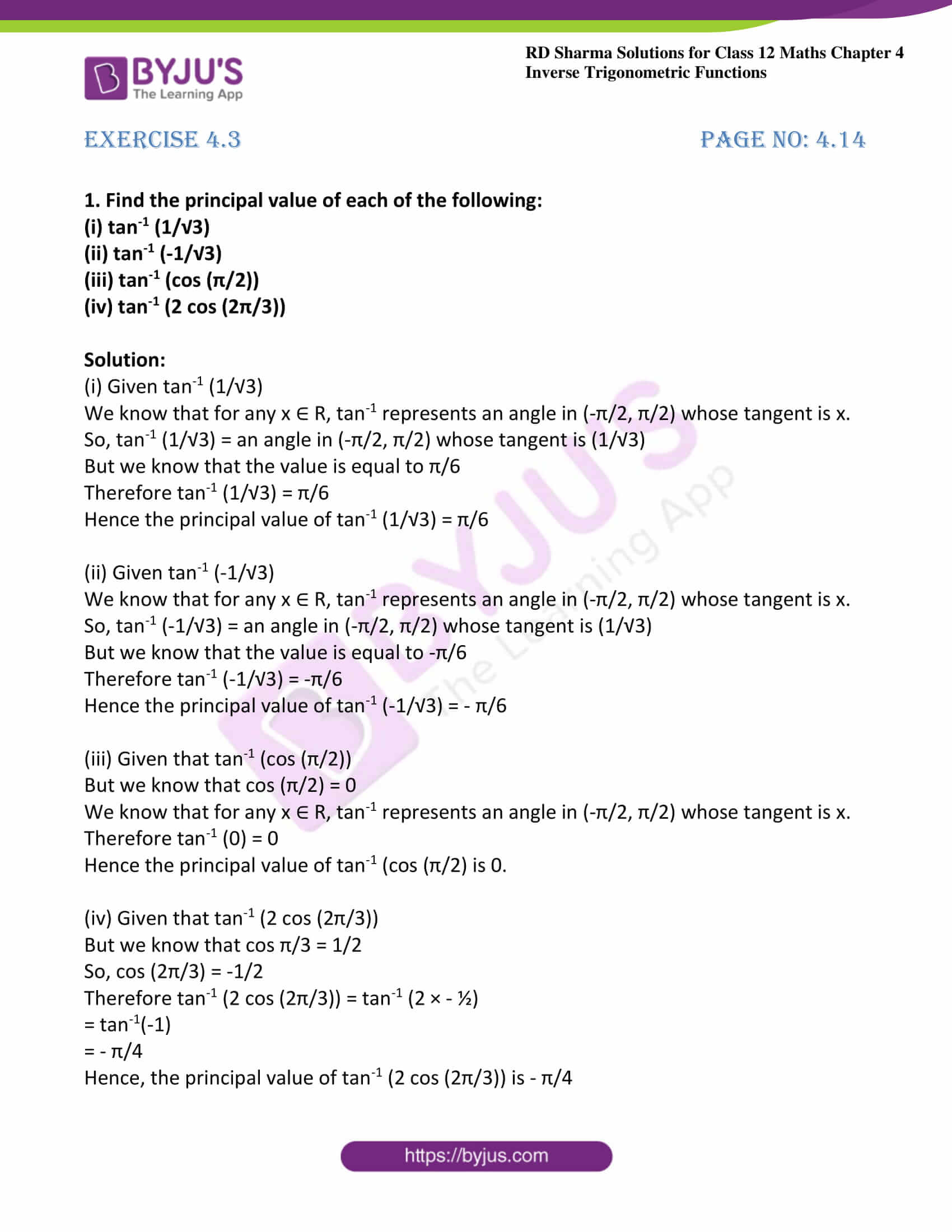# RD Sharma Solutions For Class 12 Maths Exercise 4.3 Chapter 4 Inverse Trigonometric Functions

RD Sharma Solutions Class 12 Maths Exercise 4.3 Chapter 4 Inverse Trigonometric Functions is provided here. Students are made familiar with the problems to help them analyse their knowledge about the chapter. The various steps followed in solving the problems of inverse trigonometric functions are explained in brief under exercise 4.3.

Students can primarily use the solutions as a reference guide to speed up their exam preparation. To improve self-analysing capacity, students can download RD Sharma Solutions Class 12 Maths Chapter 4 Inverse Trigonometric Functions Exercise 4.3, from the links given below.

## Download the PDF of RD Sharma Solutions For Class 12 Chapter 4 – Inverse Trigonometric Functions Exercise 4.3### Exercise 4.3 Page No: 4.14

1. Find the principal value of each of the following:

(i) tan-1 (1/√3)

(ii) tan-1 (-1/√3)

(iii) tan-1 (cos (π/2))

(iv) tan-1 (2 cos (2π/3))

Solution:

(i) Given tan-1 (1/√3)

We know that for any x ∈ R, tan-1 represents an angle in (-π/2, π/2) whose tangent is x.

So, tan-1 (1/√3) = an angle in (-π/2, π/2) whose tangent is (1/√3)

But we know that the value is equal to π/6

Therefore tan-1 (1/√3) = π/6

Hence the principal value of tan-1 (1/√3) = π/6

(ii) Given tan-1 (-1/√3)

We know that for any x ∈ R, tan-1 represents an angle in (-π/2, π/2) whose tangent is x.

So, tan-1 (-1/√3) = an angle in (-π/2, π/2) whose tangent is (1/√3)

But we know that the value is equal to -π/6

Therefore tan-1 (-1/√3) = -π/6

Hence the principal value of tan-1 (-1/√3) = – π/6

(iii) Given that tan-1 (cos (π/2))

But we know that cos (π/2) = 0

We know that for any x ∈ R, tan-1 represents an angle in (-π/2, π/2) whose tangent is x.

Therefore tan-1 (0) = 0

Hence the principal value of tan-1 (cos (π/2) is 0.

(iv) Given that tan-1 (2 cos (2π/3))

But we know that cos π/3 = 1/2

So, cos (2π/3) = -1/2

Therefore tan-1 (2 cos (2π/3)) = tan-1 (2 × – ½)

= tan-1(-1)

= – π/4

Hence, the principal value of tan-1 (2 cos (2π/3)) is – π/4

### Access other exercises of RD Sharma Solutions For Class 12 Chapter 4 – Inverse Trigonometric Functions

Exercise 4.1 Solutions

Exercise 4.2 Solutions

Exercise 4.4 Solutions

Exercise 4.5 Solutions

Exercise 4.6 Solutions

Exercise 4.7 Solutions

Exercise 4.8 Solutions

Exercise 4.9 Solutions

Exercise 4.10 Solutions

Exercise 4.11 Solutions

Exercise 4.12 Solutions

Exercise 4.13 Solutions

Exercise 4.14 Solutions